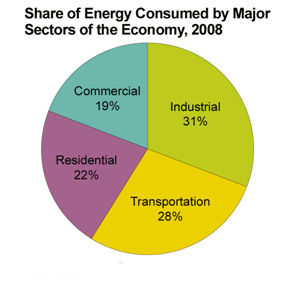Motor controls
Home » Blog » Motor controls » Output torque of variable speed drive running above 50Hz

# Output torque of variable speed drive running above 50Hz

Generally, electric motors are designed according to 50Hz power supply, its rated torque also in this frequency. Therefore, the speed adjustment under rated frequency called constant torque speed adjustment. (T = Te, P <= Pe).

If the variable speed drive outputs frequency exceeds 50Hz, the motor torque is inversely proportional to the frequency in linear relationship decrease.
When the motor running in above 50Hz frequency, we should consider the motor loads to avoid motor lacks of torque.

For example, the motor torque is about a half in 100Hz running against 50Hz. Therefore, the speed adjustment in above rated frequency called constant power speed adjustment. (P = Ue * Ie).

As we know, for a specified motor, the rated voltage and rated current is constant.

For example, the variable speed drive and motor rated values are: 15kW/380V/30A, motors can operate at 50Hz or above.
When the frequency is 50Hz, the variable speed drive output voltage is 380V, current is 30A. Then if we increase the output frequency to 60Hz, the variable speed drive maximum voltage and current also is 380V/30A, it is obviously that the output power is fixed, so it called constant power speed adjustment, what's the torque status now?

Since P = wT (w: angular speed, T: torque), as P keeps same, w increases, so the torque will decrease accordingly.

From another point: motor stator voltage U = E + I * R (I is the current, R is the electrical resistance, E is the EMF)
Then we can see, U and I are constant, E is constant.
And E = k * f * X, (k: constant, f: frequency, X: flux), when f changes from 50 to 60Hz, X will decrease accordingly.

For the motor, T = K * I * X, (K: constant, I: current, X: flux), so the torque T will decrease along with the flux X.

And, if the frequency is less than 50Hz, as I * R is very small, so if the U/f = E/f is constant, the magnetic flux (X) is constant, the torque is proportional to the current, which is why use the variable speed drive overcurrent capability to describe its overload (torque) capability, and known as constant torque speed adjustment (rated current is constant -> Maximum torque is constant).

Conclusion: When the variable speed drive outputs frequency increases from 50Hz, the motor outputs torque will decrease.

Calculate (1 * 7) =

You may also like:

Variable frequency drives are electronic devices, they have stringent requirements in installation environment which is specified in its user manual normally. In exceptional circumstances, if it does not meet ...
Stiff voltage sources are not problematic as long as they don't get in the way of the solver's attempts to linearize the behavior of the circuit matrix via step size reduction. It is the highly nonlinear stiff ...
First, specify that this is an isolated system with two generators feeding the same bus. Operation of an isolated system is different than a grid connected system, and the mode setting of the governors have to ...
Overload protection: the soft starter has current control loop to track and detect of the changes of the electric motor current. Achieve overload protection by increasing overload current settings and inverse ...
It depends on so much more than the simple requirements listed of high starting torque and variable speed. What kind of application are you using it for? Is it on an automobile (where you have DC already), a ...Gozuk Blog: all about electric motor control & drives industries development in energy saving applications.

Featured

Like pumps, fans consume significant electrical energy while serving several applications. In many plants, the VFDs (variable ...A frequency inverter controls AC motor speed. The frequency inverter converts the fixed supply frequency (60 Hz) to a ... Motor starter (also known as soft starter, motor soft starter) is a electronic device integrates soft start, soft stop, ... Soft starter allows the output voltage decreases gradually to achieve soft stop, in order to protect the equipment. Such as the ... Soft Starter reduces electric motor starting current to 2-4 times during motor start up, reduces the impact to power grid during ...

In Discussion